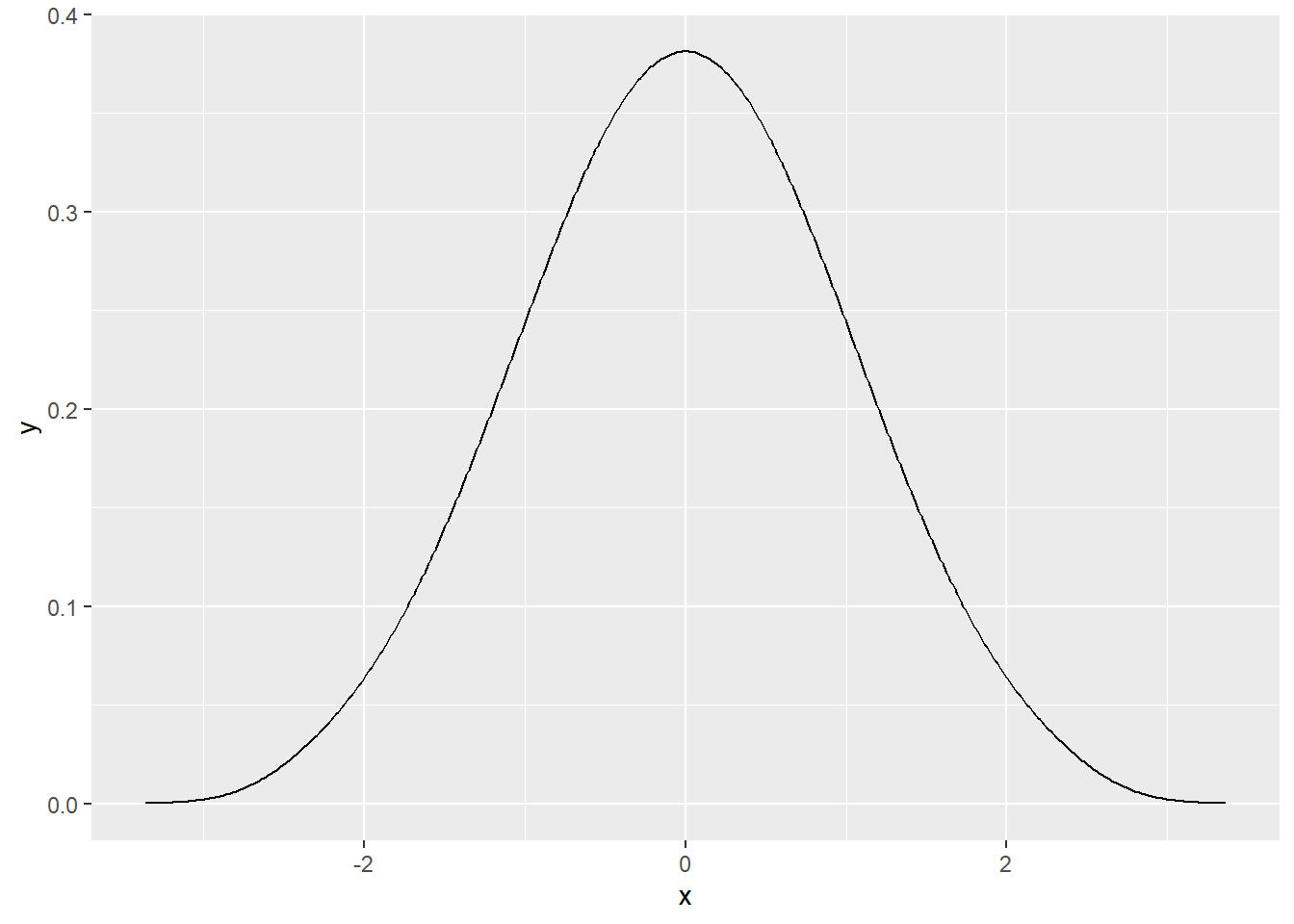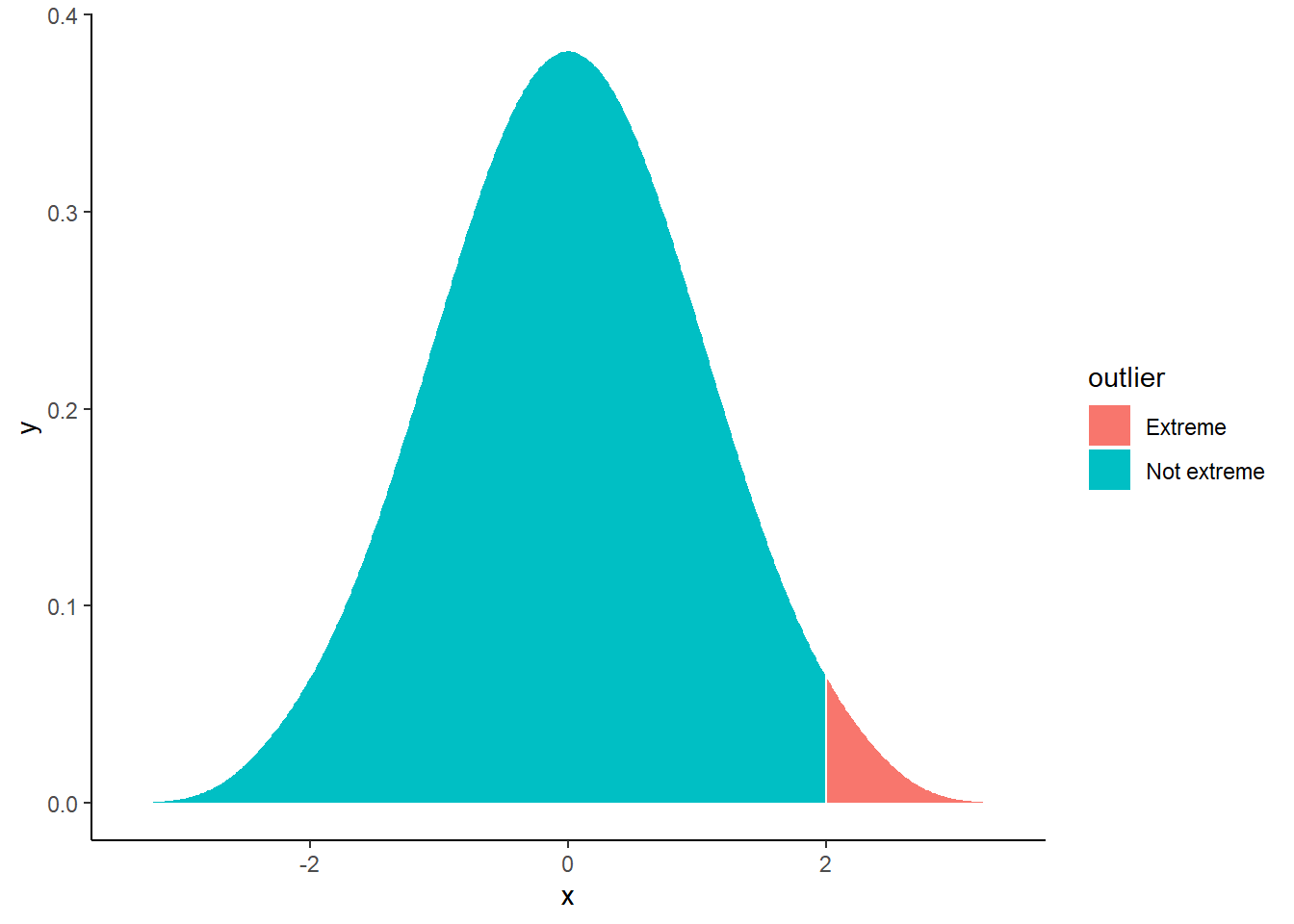# How to easily generate a perfectly normal distribution

Many times, for instance when teaching, I needed to quickly and simply generate a perfectly normally distributed sample to illustrate or show some of its characteristics.

This is now very easy to do with the new `bayestestR` package, which includes the `rnorm_perfect` function. This function is very similar to the classic `rnorm` (same arguments), with the difference that the generated sample is perfectly normal.

## Example

`bayestestR` can be installed as follows:

``````install.packages("bayestestR")  # Install the package
``````# Generate a perfect sample
x <- rnorm_perfect(n = 100, mean = 0, sd = 1)

# Visualise it
library(tidyverse)

x %>%
density() %>%  # Compute density function
as.data.frame() %>%
ggplot(aes(x=x, y=y)) +
geom_line()``````We can also easily color some of the parts of the curve, for instance, the observations lying beyond +2 standard deviations.

``````x %>%
density() %>%  # Compute density function
as.data.frame() %>%
mutate(outlier = ifelse(x > 2, "Extreme", "Not extreme")) %>%
ggplot(aes(x=x, y=y, fill=outlier)) +
geom_ribbon(aes(ymin=0, ymax=y)) +
theme_classic()``````## bayestestR and easystats

More details about `bayestestR`’s features are comming soon, stay tuned ;)

Feel free to let us know how we could further improve this package! Also, note that easystats, the project supporting `bayestestR` is in active development. Thus, do not hesitate to contact us if you want to get involved :)

• Check out our other blog posts here!